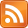[Epistemic Status: I'm just writing this to help myself remember. YMMV.]

Question: we can write down a general formula for the roots of a quadratic, cubic, or quartic polynomial in terms of the coefficients. Why can't we do it for a quintic polynomial?

Explanation: Consider the field $\mathbb{Q}(\sqrt{2})$ which is the rationals extended with $\sqrt{2}$. Consider automorphisms of this field which leave the subfield $\mathbb{Q}$ fixed. Denote by $\text{Gal}(\mathbb{Q}(\sqrt{2}) / \mathbb{Q})$ the group of these automorphisms. In this example there is one nontrivial automorphism, which takes $a + b\sqrt{2}$ to $a - b\sqrt{2}$ and vice versa. So the Galois group of $\mathbb{Q}(\sqrt{2})$ over $\mathbb{Q}$ is $C_2$.

Define the splitting field of a polynomial in a field $F$ as the smallest field extension of $F$ in which that polynomial factors. Use the notation $\text{Split}(p)$ which I made up. For example, $x^2 - 2 = (x - \sqrt{2})(x + \sqrt{2})$, so $\text{Split}(x^2 - 2)$ is $\mathbb{Q}(\sqrt{2})$.

The roots of a polynomial $p$ must live in the splitting field of $p$.

Fundamental Theorem of Galois Theory: For a field $F$ which is an extension of $\mathbb{Q}$, there is an isomorphism between the field extensions that build from $\mathbb{Q}$ up to $F$ and chains of normal subgroups $(N_1 \le N_2 ~\text{...}~ \le \text{Gal}(F/\mathbb{Q}))$ such that $N_1$ is a normal subgroup of $N_2$, $N_2$ is a normal subgroup of $N_3$, etc.

Here's an example for the polynomial $(x^2 - 2)(x^2 - 3)$, with a chain three elements long. Note that the left and right diagrams are the reverses of each other, in the sense that the "trivial" field case corresponds to the "full" group case, and vice versa. In the diagram on the right, $f$ and $g$ are generators with $f^2 = g^2 = (fg)^2 = e$.$f$ takes $a + b\sqrt{3}$ to $a - b\sqrt{3}$, $g$ takes $a + b\sqrt{2}$ to $a - b\sqrt{2}$, and $fg$ takes $a + b\sqrt{3}\sqrt{2}$ to $a - b\sqrt{3}\sqrt{2}$.

The groups $S_2, S_3, S_4$ all have the property of decomposing into chains of normal subgroups in this way. But $S_{\geq 5}$ does not have this property (not "solvable")*.

Claim: We can write down the roots of a polynomial $p$ using (iterated) radicals if and only if $\text{Gal}(\text{Split}(p))$ is solvable.

This is true because of the isomorphism between field extensions and subgroups. We build a tower of field extensions (by adding roots) which culminate in a field that can hold the roots of our polynomial. In parallel, we descend a chain of normal subgroups.

But there is no such chain for $S_{\geq 5}$, because it isn't solvable. Hence for a polynomial like $x^5 - x + 2$, which has Galois group $S_5$, we can't write its roots down in terms of (iterated) radicals. Not all polynomials of degree 5 have Galois group $S_5$, but this one does.

Because we can't descend the chain of normal subgroups, we can't by adding radicals build the field required to hold the roots of this polynomial. These roots have symmetries which are too richly structured to get at in this way.

* The obstruction is that $A_5$, the only candidate to start the chain off with, doesn't have any normal subgroups (it is "simple").

Extra:

• Implication which I somehow never realized until right now: there exist algebraic numbers, i.e. the roots of some $p \in \mathbb{Q}[x]$, which we have no "formula for". That is, no agreed-upon way to write them other than just exhibiting $p$. (You might object that this is a matter of convention and no different than the situation with $\sqrt{2}$, and you might have a point.) At any rate, these are called non-solvable numbers.

[Edited 12/9/2019. Elaborated and removed an incorrect use of the $\text{Gal}$ function.]